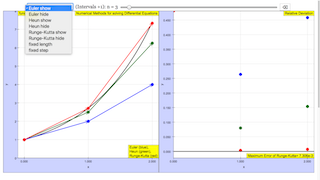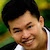Description

# numerical solution

### Comparison of Euler, Heun und Runge−Kutta algorithms

In this simulation the code for the three above mentioned algorithms is demonstrated, as applied to the numerical solution of an explicit, linear ordinary differential equation of first order. Starting from an initial value, a series of consecutive points is calculated and shown in a graphic.

As an example the exponential function is chosen with

differential equation dy/dt  = y ´ = y

analytic solution y (t) = (initial value) * et

For this special function the differential equation does not explicitly depend on the variable t, which allows a simple graphical interpretation of the approach.

When opening the file the abscissa range is divided into 5 intervals. The number of intervals can be chosen between 1 and 24 by means of a slider; the interval widths change correspondingly.

The default initial value for x = 0 is y = 1 . It can be changed with a second slider. The analytic solution curve (blue) changes with y0 . Each change starts a new calculation of the numerical approximations for the three methods.

The discrete points calculated with Euler are drawn blue, those with Heun in green, those with Runge_Kutta (4 step) in red.

Back resets the number of intervals and initial value.

A second window shows the relative residual difference between the numerical approximations and the analytic solution. Coordinate scales adjust themselves to the mistake of the Euler approach, for which it is largest.

When you open this file from the EJS console you can easily insert other differential equations instead of the very simple one of the exponential. The corresponding places are highlighted in the code pages.

Code

Just a few lines of code are sufficient for the numeric algorithms. They are applied in a loop as often as there are intervals. The end value of one loop is the initial value for the next one.

Parameters that are constant for all methods:

Initial value (for x = 0)

Range of Variables

Number of intervals n

Width of intervals delta = Range/(n-1)

Variables x , y, first derivative (derivative)

specified for the methods by subscript, as xE, xH, xRK

Runge−Kutta uses intermediate values of the first derivative, which are specified by subscripts a und b (derivative_a, derivative_b)

Loops have an index i that runs from 0 to n, as xE[i]

F[i] are deviations of the numerical results from the analytic ones.

Euler & Heun

Euler

You will find this code in the EJS console at page Initialization.

lines marked //  include remarks that do not influence the calculation.

xE=0;

yE=valueFrom;

derivativeE=yE;//for exponential

for (var i=1; i<n; i++) {//loop definitionn)

xE[i]=xE[i-1]+delta;

yE[i]=yE[i-1]+delta*derivativeE[i-1];

derivativeE[i]=yE[i];//for exponential

//back to start of loop

FE[i]=(valueFrom*Math.exp(xE[i])-yE[i])/(valueFrom*Math.exp(xE[i]));

}

Heun

xH=0;

yH=valueFrom;

derivativeH_a=yH;//for exponential

for (var i=1; i<n; i++) {//loop definition)

xH[i]=xH[i-1]+delta;

yH_a[i]=yH[i-1]+delta*derivativeH_a[i-1];

derivativeH_b[i]=yH_a[i];////for exponential

yH[i]=yH[i-1]+delta/2*(derivativeH_a[i-1]+derivativeH_b[i]);

derivativeH_a[i]=yH[i];////for exponential

//back to loop

FH[i]=(valueFrom*Math.exp(xH[i])-yH[i])/(valueFrom*Math.exp(xH[i]));

}

Runge-Kutta_Code 2

Runge-Kutta 4 steps

You will find this code in the EJS console at page Initialization

lines marked //  include remarks that do not influence the calculation.

xRK=0;

yRK=valueFrom;

derivativeRK=yRK;//for exponential

for (var i=1; i<n; i++) { //loop definition)

y_a[i]=yRK[i-1]+delta/2*derivativeRK[i-1];

derivative_a[i]=y_a[i];//for exponential

y_b[i]=yRK[i-1]+delta/2*derivative_a[i];

derivative_b[i]=y_b[i];//for exponential

y_c[i]=yRK[i-1]+delta*derivative_b[i];

derivative_c[i]=y_c[i];//for exponential

yRK[i]=yRK[i-1]+delta/6*(derivativeRK[i-1]+2*derivative_a[i]+2*derivative_b[i]+derivative_c[i]);

xRK[i]=xRK[i-1]+delta;

derivativeRK[i]=yRK[i];//for exponential

FRK[i]=(valueFrom*Math.exp(xRK[i])-yRK[i])/(valueFrom*Math.exp(xRK[i]));

//back to start of loop

}

Experiments

E 1: Choose the number of intervals as n = 5. Visually compare the quality of the three numerical approximations. Use the right window with its zoomed scale for that purpose, too.

E 2: Change the number of intervals n and compare qualitatively how the three approximations approach the analytic solution.

E 3: Make notes of the relative mistakes of the three methods with increasing n and draw a graph of their dependences. Compare this graph with a straight line for Euler and with a second grade parabola for Heun (with Runge−Kutta the deviations will be too small to clearly recognize the graph as a fourth grade parabola).

E 4: Open the file from the EJS console and study the code at page Initialization. Use other derivatives to solve different differential equations (for the exponential the derivative was simply y). The correct places are marked in red.

Authors

created by Dieter Roess in March 2009

This simulation is part of

– Plus 2000 Examples from Physics”

ISBN 978-3-11-025005-3, Walter de Gruyter GmbH & Co. KG

### Translations

Code Language Translator Run### Software Requirements

SoftwareRequirements

 Android iOS Windows MacOS with best with Chrome Chrome Chrome Chrome support full-screen? Yes. Chrome/Opera No. Firefox/ Samsung Internet Not yet Yes Yes cannot work on some mobile browser that don't understand JavaScript such as..... cannot work on Internet Explorer 9 and below

### CreditsDieter Roess - WEH- Foundation; Tan Wei Chiong; Loo Kang Wee

[text]

### For Teachers

This simple simulation helps to visualize the different numerical methods of solving differential equations.

The three methods that this simulation provides are the Euler method, the Heun method (otherwise known as the Improved Euler method), and the Runge-Kutta method (specifically RK4).

There are two graphs shown in the simulation. The one of the left shows visually how well each method approximates the equation y = e^x, while the graph on the right shows the relative deviation of each method from the actual curve formed by y = e^x

Each numerical method of solving the differential equation y' = y is color-coded as shown on both graphs.

The number of intervals (steps) can be adjusted with the slider at the top, going up to n = 25. The button at the top right resets the simulation.

As the number of intervals increase, note how the approximations become more accurate to the original curve.

Research

[text]

[text]

### Version:

1. http://weelookang.blogspot.sg/2016/02/vector-addition-b-c-model-with.html improved version with joseph chua's inputs
2. http://weelookang.blogspot.sg/2014/10/vector-addition-model.html original simulation by lookang

[text]

### end faq